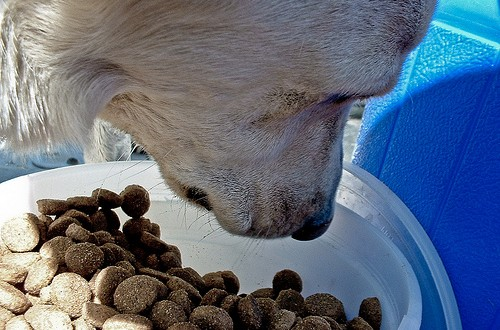# 破解寵物食品宣傳的陷阱-如何計算寵物食品的碳水化合物比例

M：寵物食品的代謝能量，也就是寵物食品每克含有的熱量，如果食品包裝袋上是每公斤熱量，則將熱量數字除以1000（例如飼料袋上的代謝能量數字是每公斤3350仟卡，則M=3.35），如果包裝袋上是每百克熱量，則將熱量數字除以100。

P：寵物食品的蛋白質比例，單位是％

F：寵物食品的油脂比例，單位是％

C：寵物食品的碳水化合物比例

1000M=(P*1000*3.5)+(C*1000*3.5)+(F*1000*8.5)

M=(P*3.5)+(C*3.5)+(F*8.5)

3.5C=M-(P*3.5)+(F*8.5)

C=((M-(P*3.5)-(F*8.5))/3.5

C
=(M-(P*3.5)-(F*8.5))/3.5

=(3.35-(0.26*3.5)-(0.06*8.5))/3.5

=(3.35-0.91-0.51)/3.5

=1.93/3.5

=55.14%

### 關於 Karen Lu